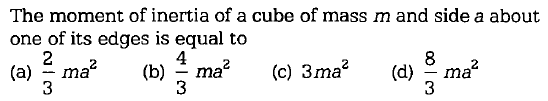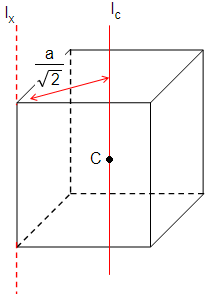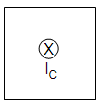# NEET Questions SolvedFirst let us find M.I. about axis $\left({\mathrm{I}}_{\mathrm{C}}\right)$ passing through center of cube.
For a square sheet, M.I. about center axis is $\frac{{\mathrm{Ma}}^{2}}{6}$ where M is mass of sheet.A cube can be assumed to be made up of infinite square sheets.
$\therefore$ For cube, moment of inertia about center $=\frac{{\mathrm{ma}}^{2}}{6}$

Let us try now to find moment of inertia about edge ${\mathrm{I}}_{\mathrm{x}}$
Using parallel-axis theorem,

Difficulty Level:

• 49%
• 20%
• 17%
• 16%